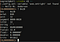xorpd has some riddle-like pieces of assembly code here. In this post, I’ll analyze this one.

I present to you this marvelous one-line riddle:

xor      al,0x20

What can we infer from this?

1. It’s using al register: a single byte it’s being used
2. 0x20 = 32 = 00100000checking the values equivalences using r2

Now, let’s talk about xor a little. This is its truth table:

input1 | input2 | result
0 | 0 | 0
0 | 1 | 1
1 | 0 | 1
1 | 1 | 0

Analyzing the second column we can see that a 0 placed there will preserve the value of input2 and a 1 will flip its value instead:

input1 | input2 | result
0 | 0 | 0 ==> input2=0, input1=result
0 | 1 | 1 ==> input2=1, input1=!result
1 | 0 | 1 ==> input2=0, input1=result
1 | 1 | 0 ==> input2=1, input1=!result

That’s why xor {regX},{regX} is used a lot by compilers to zero out a register. Observe that any value XORed with itself is equal to 0. i.e: the operation 00110101 xor 00110101 can be expressed like:

input1 | input2 | input1 xor input2
0 | 0 | 0
0 | 0 | 0
1 | 1 | 0
1 | 1 | 0
0 | 0 | 0
1 | 1 | 0
0 | 0 | 0
1 | 1 | 0
Basically every 0 is preserved and every 1 is flipped so we end up with all zeros as results

So, going back to our riddle. We can express what we know so far as:

al   |   0x20 | xor al, 020
? | 0 | ?
? | 0 | ?
? | 1 | x ==> this bit will be flipped
? | 0 | ?
? | 0 | ?
? | 0 | ?
? | 0 | ?
? | 0 | ?
As the only 1 in 0x20 is at bit n°6 that's the only bit that will flipped on al

How does this bit flip affects al? It will add or subtract 32 (0x20) from al depending on al’s original value:

Case 1: Imagine al to be 0x0 = 0 = 00000000
al | 0x20 | xor al, 020
0 | 0 | 0
0 | 0 | 0
0 | 1 | 1
0 | 0 | 0
0 | 0 | 0
0 | 0 | 0
0 | 0 | 0
0 | 0 | 0
result = 0x20 = 32 = 00100000
Case 2: Imagine al to be 0xff = 255 = 11111111
al | 0x20 | xor al, 020
1 | 0 | 1
1 | 0 | 1
1 | 1 | 0
1 | 0 | 1
1 | 0 | 1
1 | 0 | 1
1 | 0 | 1
1 | 0 | 1
result = 0xdf = 223 = 11000000

Let’s try some more values:

al   | 0x20 | xor al, 020
0x00 | 0x20 | 0x20 = 00100000 = 32 = " "
0xff | 0x20 | 0xdf = 11011111 = 223 = (no ascii value)
0x41 | 0x20 | 0x61 = 01100001 = 97 = "a"
0x50 | 0x20 | 0xdf = 01110000 = 112 = "p"
0x61 | 0x20 | 0x41 = 01000001 = 65 = "A"

From this, it’s clear that values from ASCII letters in uppercase are converted to its lowercase counterpart and vice-versa. We can check this by calculating the distance between the boundary characters:

Upper | lower | distance
A | a | 0x41 - 0x61 = 0x20
Z | z | 0x5a - 0x7a = 0x20

So, long story short this single line of assembly performs a lowercase/uppercase ASCII conversion! isn’t that awesome?

Written by

Syscall59 — by Alan Vivona

Shellcode for the masses

Welcome to a place where words matter. On Medium, smart voices and original ideas take center stage - with no ads in sight. Watch
Follow all the topics you care about, and we’ll deliver the best stories for you to your homepage and inbox. Explore
Get unlimited access to the best stories on Medium — and support writers while you’re at it. Just \$5/month. Upgrade